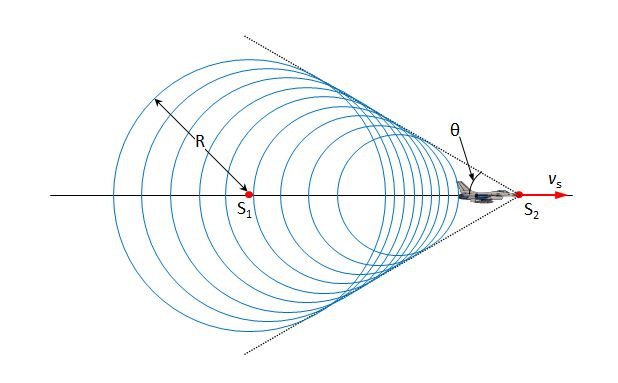# Shock Wave Of Jet PlaneThe above is a schematic diagram of the shock wave of a jet plane (the sound source) which is flying at a constant velocity of $v_s.$ When the sound source moves from $S_1$ to $S_2$, the sound wave which was generated at $S_1$ progresses a distance of $R$ as above. Which of the following explanations is correct? (The velocity of sound is $v$.)

a) $v_s$ is larger than $v$.

b) The distance between $S_1$ and $S_2$ is $\frac{v_sR}{v}$.

c) If $v_s$ becomes bigger, then $\theta$ becomes bigger, too.

×